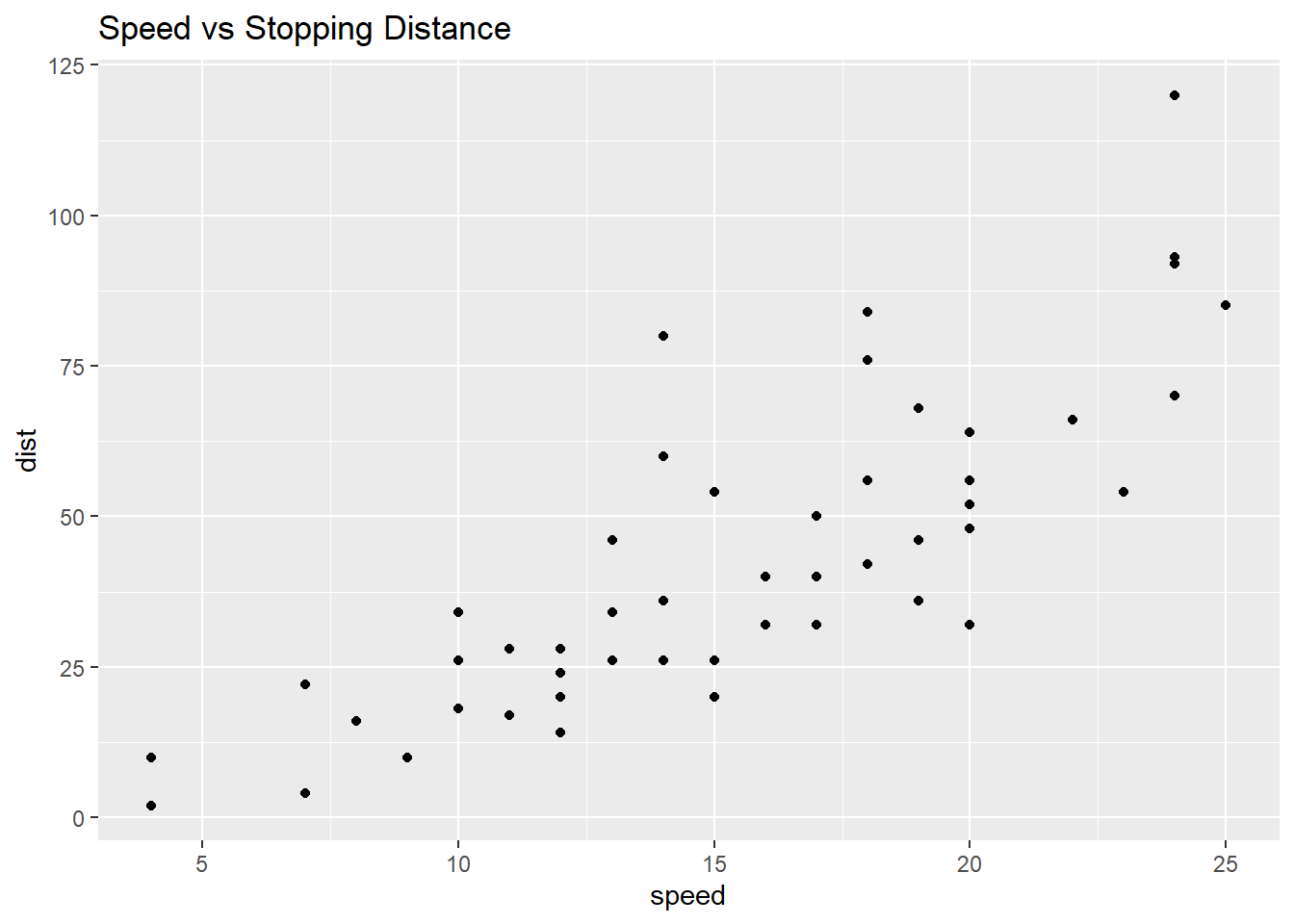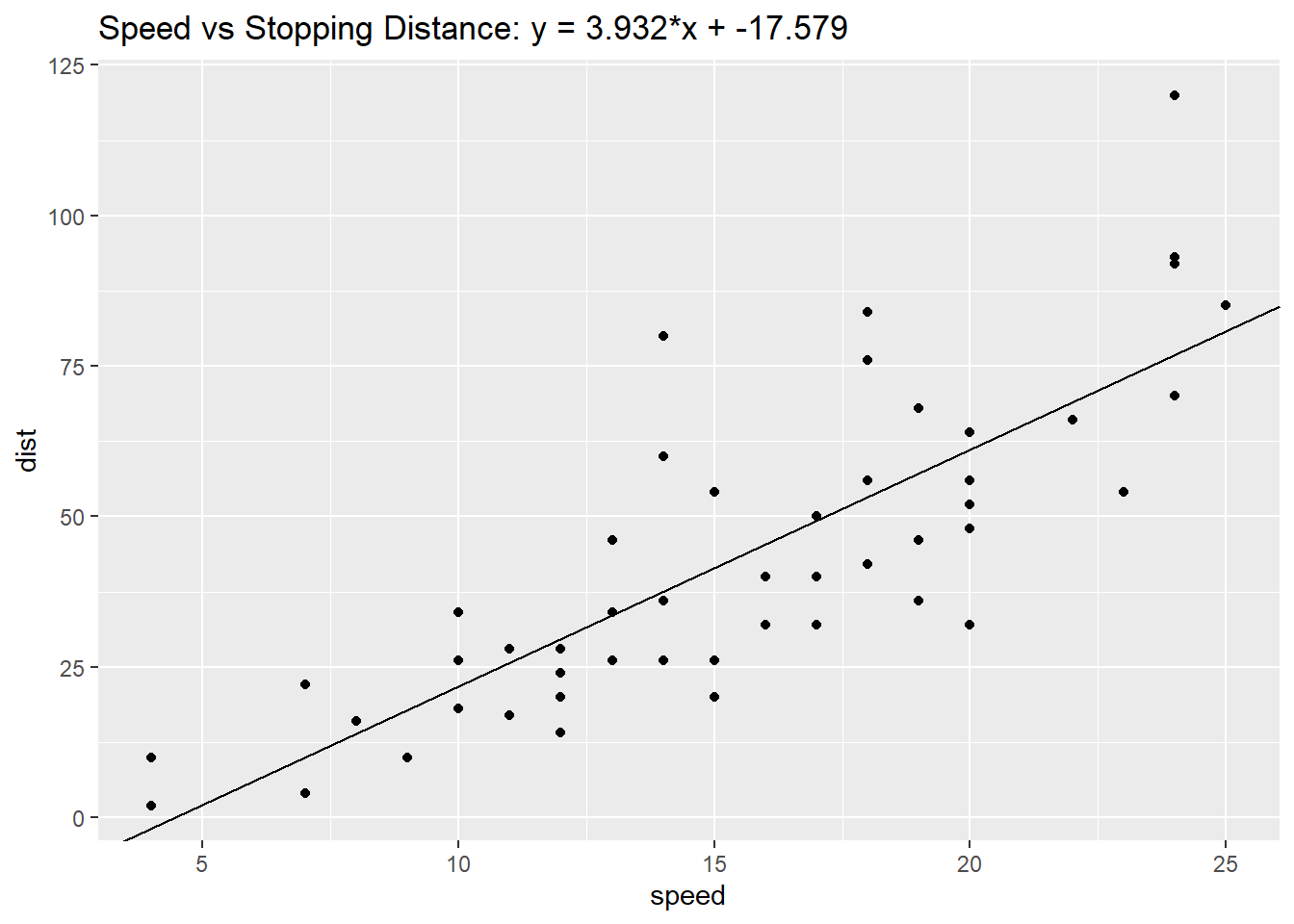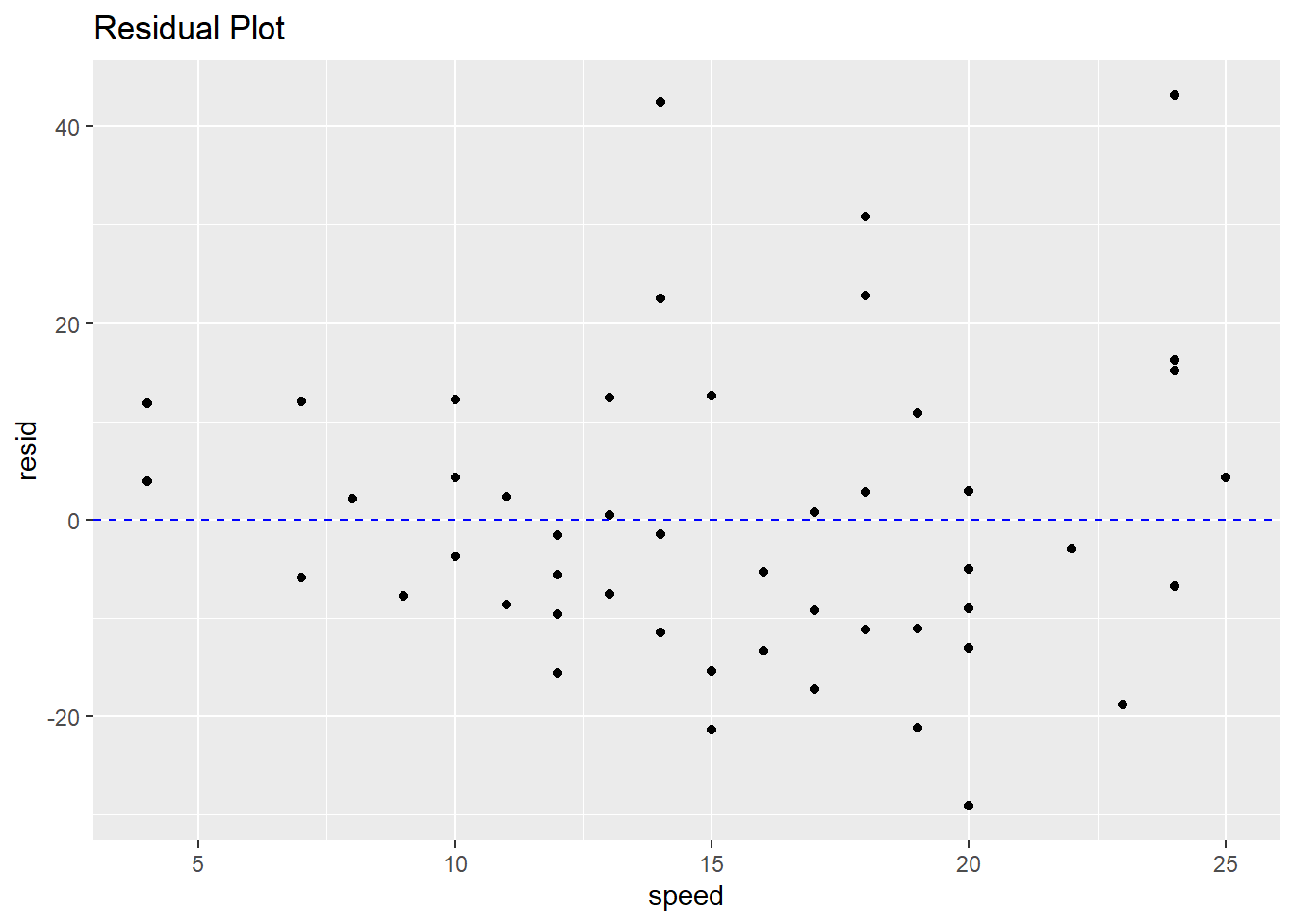# Regression - HW 11

## Cars - With Outliers

Using the “cars” dataset in R, build a linear model for stopping distance as a function of speed and replicate the analysis of your textbook chapter 3 (visualization, quality evaluation of the model, and residual analysis.)

data(cars)
head(cars, 6)
##   speed dist
## 1     4    2
## 2     4   10
## 3     7    4
## 4     7   22
## 5     8   16
## 6     9   10

### Visualization

A scatter plot of stopping distance as a function of speed.

ggplot(cars, aes(speed,dist)) + geom_point() +
ggtitle("Speed vs Stopping Distance")### Linear Model

attach(cars)
lm <- lm(dist ~ speed)
lm
##
## Call:
## lm(formula = dist ~ speed)
##
## Coefficients:
## (Intercept)        speed
##     -17.579        3.932
eq = paste0("y = ", round(lm$coefficients,3), "*x + ", round(lm$coefficients,3))
ggplot(cars, aes(speed,dist)) + geom_point() +
geom_abline(intercept = lm$coefficients, slope = lm$coefficients) +
ggtitle(paste("Speed vs Stopping Distance:",eq))### Model Quality

• This model has an intercent of 17.5791 and a slope of 3.9324, so for every additional unit of speed, the stopping distance increases by 3.9324.
• The model has 48 degree of freedom, which is the number of samples minus the number of coefficents in the model (50 - 2).
• To determine the model quality, we look at the Multiple R-squared value. R-squared values closer to one indicate better model quality, so R^2 = 0.6511 indicates that this model is sufficient.
summary(lm)
##
## Call:
## lm(formula = dist ~ speed)
##
## Residuals:
##     Min      1Q  Median      3Q     Max
## -29.069  -9.525  -2.272   9.215  43.201
##
## Coefficients:
##             Estimate Std. Error t value Pr(>|t|)
## (Intercept) -17.5791     6.7584  -2.601   0.0123 *
## speed         3.9324     0.4155   9.464 1.49e-12 ***
## ---
## Signif. codes:  0 '***' 0.001 '**' 0.01 '*' 0.05 '.' 0.1 ' ' 1
##
## Residual standard error: 15.38 on 48 degrees of freedom
## Multiple R-squared:  0.6511, Adjusted R-squared:  0.6438
## F-statistic: 89.57 on 1 and 48 DF,  p-value: 1.49e-12

### Residual Analysis

The residuals do not have a trend, which indicates that a linear model fits the data well. However, there are more residual values below 0 than above 0, which suggests that the data may have outliers.

cars$resid <- resid(lm) ggplot(cars, aes(speed,resid)) + geom_point() + geom_hline(yintercept=0, linetype="dashed", color = "blue") + ggtitle("Residual Plot")### Outliers dist_outlier <- boxplot.stats(cars$dist)\$out
print(paste("There is a stopping distance outlier at",dist_outlier))
##  "There is a stopping distance outlier at 120"